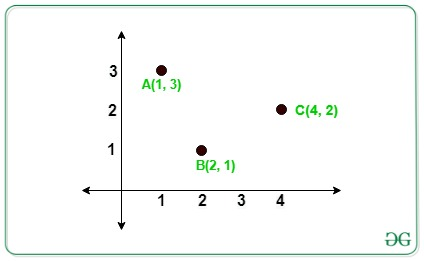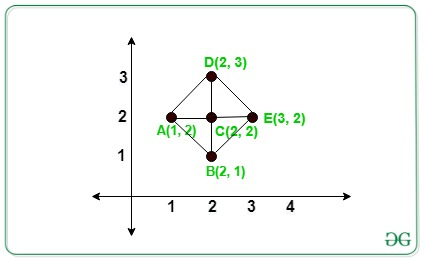# Count of Right-Angled Triangle formed from given N points whose base or perpendicular are parallel to X or Y axis

Given an array arr[] of N distinct integers points on the 2D Plane. The task is to count the number of Right-Angled Triangle from N points such that the base or perpendicular is parallel to the X or Y-axis.

Examples:

Input: arr[][] = {{4, 2}, {2, 1}, {1, 3}}
Output:
Explanation:In the above image there is no right-angled triangle formed.
Input: arr[][] = {{1, 2}, {2, 1}, {2, 2}, {2, 3}, {3, 2}}
Output:
Explanation:In the above image there are 4 right-angled triangles formed by triangles ACB, ACD, DCE, BCE.

Approach: The idea is to store the count of each co-ordinate’s having the same X and Y co-ordinates respectively. Now traverse each given points and the count of a right-angled triangle formed by each coordinate (X, Y) is given by:

Count of right-angled triangles = (frequencies of X coordinates – 1) * (frequencies of Y coordinates – 1)

Below are the steps:

• Create two maps to store the count of points, one for having the same X-coordinate and another for having the same Y-coordinate.
• For each value in the map of x-coordinate and in the map of y-coordinate choose that pair of points as pivot elements and find the frequency of that pivot element.
• For each pivot element(say pivot) in the above step, the count of right-angled is given by:

(m1[pivot].second-1)*(m2[pivot].second-1)

• Similarly, calculate the total possible right-angled triangle for other N points given.
• Finally, sum all the possible triangle obtained that is the final answer.

Below is the implementation of the above approach:

## C++

 `// C++ program for the above approach ` ` `  `#include ` `using` `namespace` `std; ` ` `  `// Function to find the number of right ` `// angled triangle that are formed from ` `// given N points whose perpendicular or ` `// base is parallel to X or Y axis ` `int` `RightAngled(``int` `a[], ``int` `n) ` `{ ` ` `  `    ``// To store the number of points ` `    ``// has same x or y coordinates ` `    ``unordered_map<``int``, ``int``> xpoints; ` `    ``unordered_map<``int``, ``int``> ypoints; ` ` `  `    ``for` `(``int` `i = 0; i < n; i++) { ` `        ``xpoints[a[i]]++; ` `        ``ypoints[a[i]]++; ` `    ``} ` ` `  `    ``// Store the total count of triangle ` `    ``int` `count = 0; ` ` `  `    ``// Iterate to check for total number ` `    ``// of possible triangle ` `    ``for` `(``int` `i = 0; i < n; i++) { ` ` `  `        ``if` `(xpoints[a[i]] >= 1 ` `            ``&& ypoints[a[i]] >= 1) { ` ` `  `            ``// Add the count of triangles ` `            ``// formed ` `            ``count += (xpoints[a[i]] - 1) ` `                     ``* (ypoints[a[i]] - 1); ` `        ``} ` `    ``} ` ` `  `    ``// Total possible triangle ` `    ``return` `count; ` `} ` ` `  `// Driver Code ` `int` `main() ` `{ ` `    ``int` `N = 5; ` ` `  `    ``// Given N points ` `    ``int` `arr[] = { { 1, 2 }, { 2, 1 }, ` `                     ``{ 2, 2 }, { 2, 3 }, ` `                     ``{ 3, 2 } }; ` ` `  `    ``// Function Call ` `    ``cout << RightAngled(arr, N); ` ` `  `    ``return` `0; ` `} `

## Python3

 `# Python3 program for the above approach  ` `from` `collections ``import` `defaultdict ` ` `  `# Function to find the number of right  ` `# angled triangle that are formed from  ` `# given N points whose perpendicular or  ` `# base is parallel to X or Y axis  ` `def` `RightAngled(a, n): ` `     `  `    ``# To store the number of points  ` `    ``# has same x or y coordinates  ` `    ``xpoints ``=` `defaultdict(``lambda``:``0``) ` `    ``ypoints ``=` `defaultdict(``lambda``:``0``) ` `     `  `    ``for` `i ``in` `range``(n): ` `        ``xpoints[a[i][``0``]] ``+``=` `1` `        ``ypoints[a[i][``1``]] ``+``=` `1` `         `  `    ``# Store the total count of triangle  ` `    ``count ``=` `0` `     `  `    ``# Iterate to check for total number  ` `    ``# of possible triangle  ` `    ``for` `i ``in` `range``(n): ` `        ``if` `(xpoints[a[i][``0``]] >``=` `1` `and`  `            ``ypoints[a[i][``1``]] >``=` `1``): ` `             `  `            ``# Add the count of triangles  ` `            ``# formed  ` `            ``count ``+``=` `((xpoints[a[i][``0``]] ``-` `1``) ``*`  `                      ``(ypoints[a[i][``1``]] ``-` `1``)) ` `             `  `    ``# Total possible triangle ` `    ``return` `count ` ` `  `# Driver Code  ` `N ``=` `5` ` `  `# Given N points  ` `arr ``=` `[ [ ``1``, ``2` `], [ ``2``, ``1` `], ` `        ``[ ``2``, ``2` `], [ ``2``, ``3` `], ` `        ``[ ``3``, ``2` `] ] ` ` `  `# Function call ` `print``(RightAngled(arr, N)) ` ` `  `# This code is contributed by Stuti Pathak`

## Java

 `// Java program for the above approach ` `import` `java.util.*; ` `class` `GFG{ ` ` `  `// Function to find the number of right ` `// angled triangle that are formed from ` `// given N points whose perpendicular or ` `// base is parallel to X or Y axis ` `static` `int` `RightAngled(``int` `a[][], ``int` `n) ` `{ ` ` `  `    ``// To store the number of points ` `    ``// has same x or y coordinates ` `    ``HashMap xpoints  = ``new` `HashMap(); ` `    ``HashMap ypoints  = ``new` `HashMap(); ` ` `  `    ``for` `(``int` `i = ``0``; i < n; i++)  ` `    ``{ ` `        ``if``(xpoints.containsKey(a[i][``0``])) ` `        ``{ ` `            ``xpoints.put(a[i][``0``], xpoints.get(a[i][``0``]) + ``1``); ` `        ``} ` `        ``else` `        ``{ ` `            ``xpoints.put(a[i][``0``], ``1``); ` `        ``} ` `        ``if``(ypoints.containsKey(a[i][``1``])) ` `        ``{ ` `            ``ypoints.put(a[i][``1``], ypoints.get(a[i][``1``]) + ``1``); ` `        ``} ` `        ``else` `        ``{ ` `            ``ypoints.put(a[i][``1``], ``1``); ` `        ``} ` `    ``} ` ` `  `    ``// Store the total count of triangle ` `    ``int` `count = ``0``; ` ` `  `    ``// Iterate to check for total number ` `    ``// of possible triangle ` `    ``for` `(``int` `i = ``0``; i < n; i++) ` `    ``{ ` `        ``if` `(xpoints.get(a[i][``0``]) >= ``1` `&& ` `            ``ypoints.get(a[i][``1``]) >= ``1``) ` `        ``{ ` ` `  `            ``// Add the count of triangles ` `            ``// formed ` `            ``count += (xpoints.get(a[i][``0``]) - ``1``) * ` `                     ``(ypoints.get(a[i][``1``]) - ``1``); ` `        ``} ` `    ``} ` ` `  `    ``// Total possible triangle ` `    ``return` `count; ` `} ` ` `  `// Driver Code ` `public` `static` `void` `main(String[] args) ` `{ ` `    ``int` `N = ``5``; ` ` `  `    ``// Given N points ` `    ``int` `arr[][] = { { ``1``, ``2` `}, { ``2``, ``1` `}, ` `                    ``{ ``2``, ``2` `}, { ``2``, ``3` `}, ` `                    ``{ ``3``, ``2` `} }; ` ` `  `    ``// Function Call ` `    ``System.out.print(RightAngled(arr, N)); ` `} ` `} ` ` `  `// This code is contributed by Rajput-Ji`

## C#

 `// C# program for the above approach ` `using` `System; ` `using` `System.Collections.Generic; ` `class` `GFG{ ` ` `  `    ``// Function to find the number of right ` `    ``// angled triangle that are formed from ` `    ``// given N points whose perpendicular or ` `    ``// base is parallel to X or Y axis ` `    ``static` `int` `RightAngled(``int``[, ] a, ``int` `n) ` `    ``{ ` ` `  `        ``// To store the number of points ` `        ``// has same x or y coordinates ` `        ``Dictionary<``int``, ``int``> xpoints = ``new` `Dictionary<``int``, ``int``>(); ` `        ``Dictionary<``int``, ``int``> ypoints = ``new` `Dictionary<``int``, ``int``>(); ` ` `  `        ``for` `(``int` `i = 0; i < n; i++)  ` `        ``{ ` `            ``if` `(xpoints.ContainsKey(a[i, 0]))  ` `            ``{ ` `                ``xpoints[a[i, 0]] = xpoints[a[i, 0]] + 1; ` `            ``} ` `            ``else`  `            ``{ ` `                ``xpoints.Add(a[i, 0], 1); ` `            ``} ` `            ``if` `(ypoints.ContainsKey(a[i, 1]))  ` `            ``{ ` `                ``ypoints[a[i, 1]] = ypoints[a[i, 1]] + 1; ` `            ``} ` `            ``else`  `            ``{ ` `                ``ypoints.Add(a[i, 1], 1); ` `            ``} ` `        ``} ` ` `  `        ``// Store the total count of triangle ` `        ``int` `count = 0; ` ` `  `        ``// Iterate to check for total number ` `        ``// of possible triangle ` `        ``for` `(``int` `i = 0; i < n; i++)  ` `        ``{ ` `            ``if` `(xpoints[a[i, 0]] >= 1 &&  ` `                ``ypoints[a[i, 1]] >= 1)  ` `            ``{ ` ` `  `                ``// Add the count of triangles ` `                ``// formed ` `                ``count += (xpoints[a[i, 0]] - 1) *  ` `                         ``(ypoints[a[i, 1]] - 1); ` `            ``} ` `        ``} ` ` `  `        ``// Total possible triangle ` `        ``return` `count; ` `    ``} ` ` `  `    ``// Driver Code ` `    ``public` `static` `void` `Main(String[] args) ` `    ``{ ` `        ``int` `N = 5; ` ` `  `        ``// Given N points ` `        ``int``[, ] arr = {{1, 2}, {2, 1},  ` `                       ``{2, 2}, {2, 3}, {3, 2}}; ` ` `  `        ``// Function Call ` `        ``Console.Write(RightAngled(arr, N)); ` `    ``} ` `} ` ` `  `// This code is contributed by Rajput-Ji`

Output:

`4`

Time Complexity: O(N)
Auxiliary Space: O(1)

Attention reader! Don’t stop learning now. Get hold of all the important DSA concepts with the DSA Self Paced Course at a student-friendly price and become industry ready.

My Personal Notes arrow_drop_upCheck out this Author's contributed articles.

If you like GeeksforGeeks and would like to contribute, you can also write an article using contribute.geeksforgeeks.org or mail your article to contribute@geeksforgeeks.org. See your article appearing on the GeeksforGeeks main page and help other Geeks.

Please Improve this article if you find anything incorrect by clicking on the "Improve Article" button below.